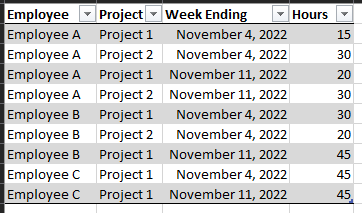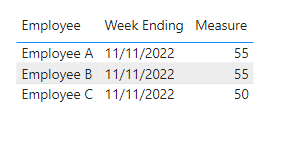cancel
Showing results for
Did you mean:Frequent Visitor

## Calculating overtime hours on a bi-weekly basis

Hello all,

I was doing some research on a solution for my problem, but still haven't come across anything that matches my scenario; however, there is this old topic that calculates overtime hours on a weekly basis based on the sample table shown in the solution.

https://community.powerbi.com/t5/Desktop/Calculating-over-time-hours-for-employees/m-p/728653

However, I would like to calculate overtime for every two weeks (bi-weekly). Here is a sample table that I will be using.Is there a way to do this or is it just not possible? I've tried so many ways and looking up information with no luck.

1 ACCEPTED SOLUTIONCommunity Support

Hi @pintobean87 ,

``````Measure =
var _a = RANKX(ALLEXCEPT('Table','Table'[Employee]),[Week Ending],MAX('Table'[Week Ending]),ASC,Dense)
var _b = MAXX(FILTER(ALLEXCEPT('Table','Table'[Employee]),[Week Ending]<MAX('Table'[Week Ending])),[Week Ending])
var _c = CALCULATE(SUM('Table'[Hours]),FILTER(ALL('Table'),[Employee]=MAX('Table'[Employee])&&[Week Ending]<=MAX('Table'[Week Ending])&&[Week Ending]>=_b))-40
return IF(_a=ODD(_a),BLANK(),_c)``````

Final output:Best Regards,

Jianbo Li

If this post helps, then please consider Accept it as the solution to help the other members find it more quickly.

5 REPLIES 5Community Support

Hi @pintobean87 ,

``````Measure =
var _a = RANKX(ALLEXCEPT('Table','Table'[Employee]),[Week Ending],MAX('Table'[Week Ending]),ASC,Dense)
var _b = MAXX(FILTER(ALLEXCEPT('Table','Table'[Employee]),[Week Ending]<MAX('Table'[Week Ending])),[Week Ending])
var _c = CALCULATE(SUM('Table'[Hours]),FILTER(ALL('Table'),[Employee]=MAX('Table'[Employee])&&[Week Ending]<=MAX('Table'[Week Ending])&&[Week Ending]>=_b))-40
return IF(_a=ODD(_a),BLANK(),_c)``````

Final output:Best Regards,

Jianbo Li

If this post helps, then please consider Accept it as the solution to help the other members find it more quickly.Frequent Visitor

I have one last question! When an employee has less than 80 hours bi-weekly, a negative number is returned. This affects the total amount of hours for all employees (since I only want to see overtime hours). Is there a way to simply not return anything if overtime is below 80?Community Support

Hi @pintobean87 ,

``````Measure =
var _a = RANKX(ALLEXCEPT('Table','Table'[Employee]),[Week Ending],MAX('Table'[Week Ending]),ASC,Dense)
var _b = MAXX(FILTER(ALLEXCEPT('Table','Table'[Employee]),[Week Ending]<MAX('Table'[Week Ending])),[Week Ending])
var _c = CALCULATE(SUM('Table'[Hours]),FILTER(ALL('Table'),[Employee]=MAX('Table'[Employee])&&[Week Ending]<=MAX('Table'[Week Ending])&&[Week Ending]>=_b))-80
return IF(_a=ODD(_a)||_c<0,BLANK(),_c)``````

Best Regards,

Jianbo Li

If this post helps, then please consider Accept it as the solution to help the other members find it more quickly.Frequent Visitor

Thank you so much! This worked great. I tried a variety of situational data, and it works perfect.Frequent Visitor

Is this not possible?